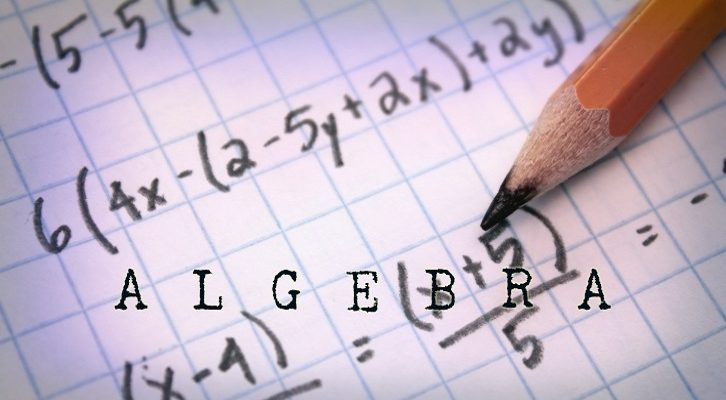# CAT – questions and solution from progressions

Have given one interesting question from progressions. This question is from challenge quiz I. This is not that difficult, but as with all good questions, there is a formula-based method of solving this and there is a more straightforward intuitive way of solving them

Question

Sum of first 12 terms of a GP is equal to the sum of the first 14 terms in the same GP. Sum of the first 17 terms is 92, what is the third term in the GP?

1. 92
2. -92
3. 46
4. 231
Correct Answer : 92. Correct Choice : (1)

Sum of first 12 terms is equal to sum of first 14 terms.
Sum of first 14 terms = Sum of first 12 terms + 13th term + 14th term
=> 13th term + 14th term = 0

Let us assume 13th term = k, common ratio = r. 14th term will be kr.
k + kr = 0
k (1 + r) = 0
=> r = -1 as k cannot be zero

Common ratio = -1.
Now, if the first term of this GP is a, second term would be -a, third would be a and so on
The GP would be a, -a, a, -a, a, -a,…

Sum to even number of terms = 0
Sum to odd number of terms = a

Sum to 19 terms is 92 => a = 92
Third term = a = 92

————————————————————————-
2iim – CAT Classes, Correspondence material.